# 发掘数据中的信息 -- 数据探索之描述性统计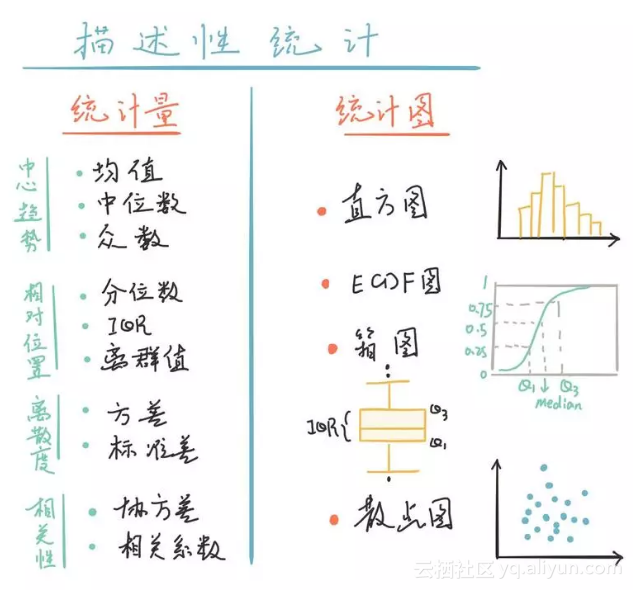（https://github.com/fishstar/Exploratory-Data-Analysis）

import pandas as pd # 导入各类模块
import numpy as np
import matplotlib.pyplot as plt
import seaborn as sns
import brfss

%config InlineBackend.figure_format = 'retina' # 设置图像清晰度
df = brfss.ReadBrfss() # 导入 BRFSS 数据

bmi_income = df[['bmi','income']].dropna() # 选取bmi和income两列数据，并舍弃缺失的数据。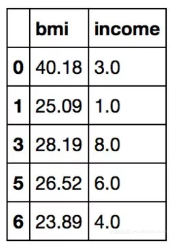bmi_income.info()

<class 'pandas.core.frame.DataFrame'>
Int64Index: 343092 entries, 0 to 441455
Data columns (total 2 columns):
bmi 343092 non-null float64
income 343092 non-null float64
dtypes: float64(2)
memory usage: 7.9 MB

bmi_rich = bmi_income[bmi_income.income == 8]['bmi']
bmi_ord = bmi_income[bmi_income.income != 8]['bmi']

describe()方法查看这两类人群的bmi数据在统计方面的信息，包括样本量（count）、均值（mean）、标准差（std）、最大（max）和最小（min）值，以及分位数。

bmi_rich.describe()

count 110259.000000
mean 27.450733
std 5.900353
min 12.050000
25% 23.690000
50% 26.570000
75% 30.040000
max 97.650000
Name: bmi, dtype: float64

bmi_ord.describe()

count 232833.000000
mean 28.537320
std 6.971436
min 12.020000
25% 24.030000
50% 27.370000
75% 31.620000
max 97.650000
Name: bmi, dtype: float64

●  均值:

mean_rich = bmi_rich.mean() # 计算均值
mean_ord = bmi_ord.mean()
print('BMI mean of rich people: %.2f' % mean_rich)
print('BMI mean of ordinary people: %.2f' % mean_ord)

●  中位数

median_rich = bmi_rich.median() # 计算中位数
median_ord = bmi_ord.median()
print('BMI median of rich people: %.2f' % median_rich)
print('BMI median of ordinary people: %.2f' % median_ord)

BMI median of rich people: 26.57
BMI median of ordinary people: 27.37

●  众数

# 计算富人的众数
mode_rich = bmi_rich.mode().iloc
mode_count_rich = np.sum(bmi_rich == mode_rich)
print('BMI mode of rich people: %.2f (counts %d)' % (mode_rich, mode_count_rich))

# 计算普通人的众数
mode_ord = bmi_ord.mode().iloc
mode_count_ord = np.sum(bmi_ord == mode_ord)
print('BMI mode of ordinary people: %.2f (counts %d)' % (mode_ord, mode_count_ord))

●  均值的差值

print("mean difference (rich - ordinary) : %.2f" % (mean_rich - mean_ord))

mean difference (rich - ordinary) : -1.09

●  直方图

fig = plt.figure(figsize=(14,4))

# 绘制富人bmi数据的直方图
plt.hist(bmi_rich, bins=50, rwidth=0.9)
plt.xlabel('BMI')
plt.ylabel('Counts')
plt.title('BMI histogram of rich people')

# 绘制普通人bmi数据的直方图
plt.hist(bmi_ord, bins=50, rwidth=0.9)
plt.xlabel('BMI')
plt.ylabel('Counts')
plt.title('BMI histogram of ordinary people') plt.show()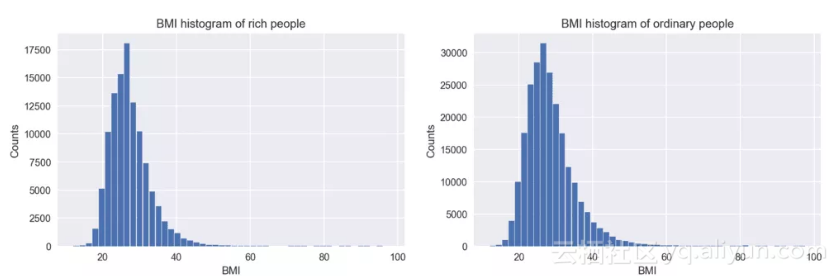plt.hist(bmi_rich, bins=50, range=(10,60), normed=True, label='rich', alpha=0.4, color='red')
plt.hist(bmi_ord, bins=50, range=(10,60), normed=True, label='ordinary', alpha=0.4, color='blue')
plt.legend()
plt.xlabel('BMI')
plt.ylabel('probability density')
plt.title('BMI histogram')
plt.show()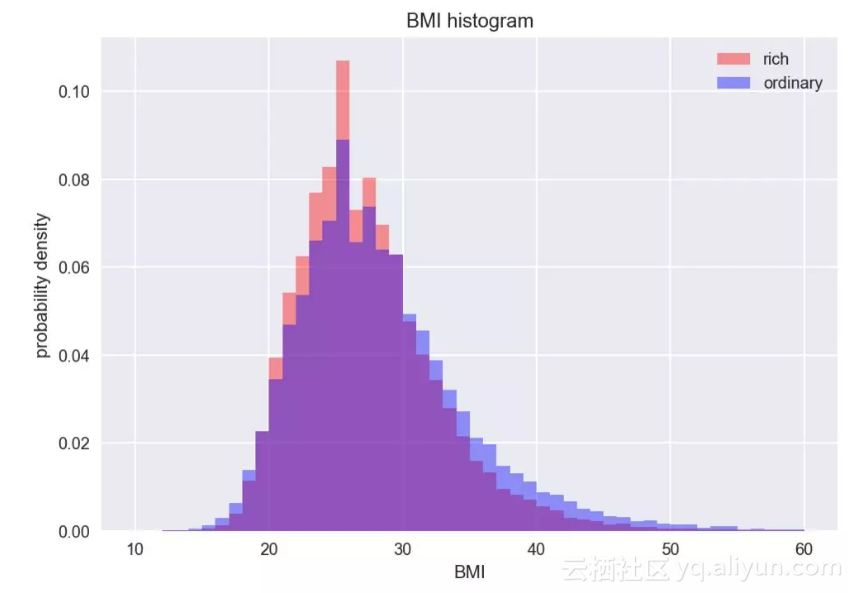●  偏度

# 计算众数区间
bin_edge = np.arange(10,60,1)
counts, bins = np.histogram(bmi_rich, bin_edge)
mode_left = bins[np.argmax(counts)]
mode_right = bins[np.argmax(counts)+1]
mode_middle = (mode_left + mode_right) / 2
print('mode range: (%.2f, %.2f)' % (mode_left, mode_right))
print('median: %.2f' % median_rich)
print('mean: %.2f' % mean_rich)

# 计算偏度
print('skewness: %.2f' %bmi_rich.skew())

# 做图
plt.axvline(x=mean_rich, linewidth=1, color='red', label='mean')
plt.axvline(x=median_rich, linewidth=1, color='green', label='median')
plt.axvline(x=mode_middle, linewidth=1, color='blue', label='mode')
plt.legend()

plt.hist(bmi_rich, bins=bin_edge, range=(10,60), rwidth=0.9, alpha=0.5)
plt.xlabel('BMI')
plt.ylabel('Counts')
plt.title('BMI distribution of rich people')
plt.show(

mode range: (25.00, 26.00)
median: 26.57
mean: 27.45
skewness: 2.58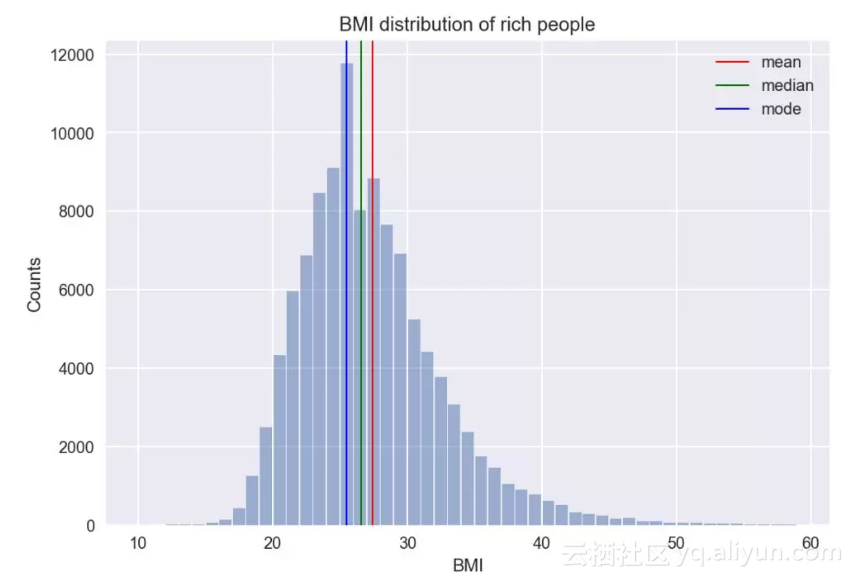# 收入水平的分布
print('skewness: %.2f' %bmi_income.income.skew())

bins = np.arange(1,10)
plt.hist(bmi_income.income, align='left', bins=bins, rwidth=0.9)
plt.title('income distribution')
plt.xlabel('income level')
plt.ylabel('counts')
plt.show()

skewness: -0.74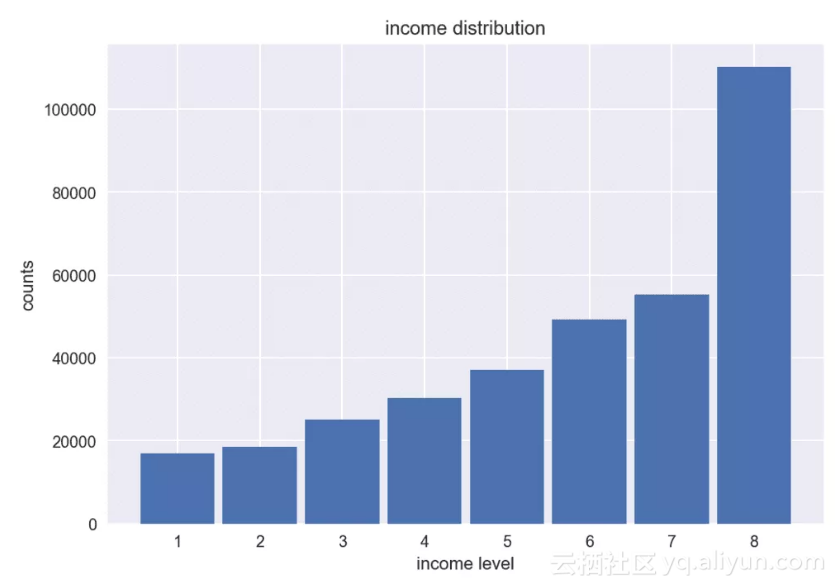●  ECDF图

def ecdf(data): # 计算数据的ECDF值
x = np.sort(data)
y = np.arange(1, len(x)+1) / len(x)
return (x,y)

def plot_ecdf(data, xlabel=None , ylabel='ECDF', label=None): # 绘制ECDF图
x, y = ecdf(data)

_ = plt.plot(x, y, marker='.', markersize=3, linestyle='none', label=label)
_ = plt.legend(markerscale=4)
_ = plt.xlabel(xlabel)
_ = plt.ylabel(ylabel)
plt.margins(0.02)

plot_ecdf(bmi_rich,label='rich')
plot_ecdf(bmi_ord, xlabel='BMI',label='ordinary')
plt.show()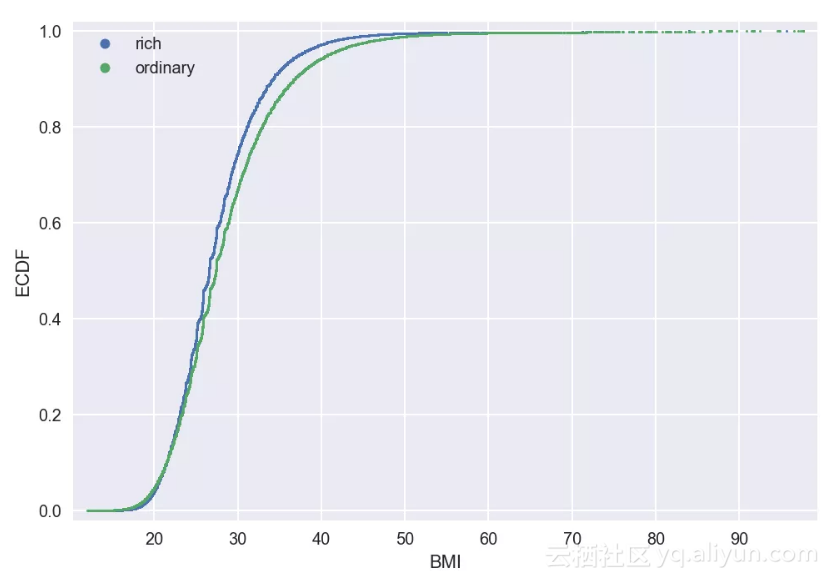ECDF图

ECDF图中显示了所有的数据点及其在样本中所处的位置，从上图中可以清晰地看到普通人群（绿色点）比富人（蓝色点）的分布更靠右，即向BMI变大的方向偏移。

●  分位数

print('min: ', bmi_rich.min())
print('max: ', bmi_rich.max())

min: 12.05
max: 97.65

q1 = bmi_rich.quantile(0.25)
q2 = bmi_rich.quantile(0.5)
q3 = bmi_rich.quantile(0.75)
IQR = q3 - q1

print('25%: ', q1)
print('50%: ', q2)
print('75%: ', q3)
print('IQR: %.2f' % IQR)

25%: 23.69
50%: 26.57
75%: 30.04
IQR: 6.35
●  箱图（box plot）

# 绘制箱图
bmi_income['income_level'] = bmi_income.income.apply(lambda x: 'rich' if x==8 else 'ordinary')
sns.boxplot(x='income_level', y='bmi', data=bmi_income, palette="Set3")
plt.show()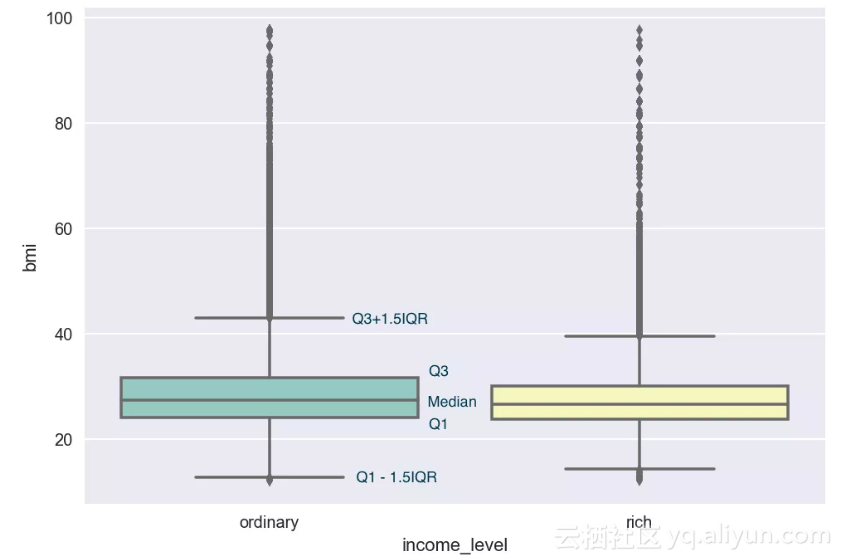●  方差和标准差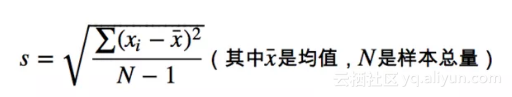var_rich = bmi_rich.var()
std_rich = bmi_rich.std()
print(' For rich people: Variance = %.2f, Standard deviation = %.2f' % (var_rich, std_rich))

var_ord = bmi_ord.var()
std_ord = bmi_ord.std()
print(' For ordinary people: Variance = %.2f, Standard deviation = %.2f' % (var_ord, std_ord))

For rich people: Variance = 34.81, Standard deviation = 5.90
For ordinary people: Variance = 48.60, Standard deviation = 6.97

●  **Cohen's d **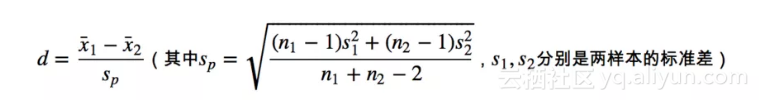Cohen's d 公式

def cohen_d(data1, data2): \
n1 = len(data1)
n2 = len(data2)
x1 = np.mean(data1)
x2 = np.mean(data2)
var1 = np.var(data1, ddof=1)
var2 = np.var(data2, ddof=1)
sp = np.sqrt(((n1-1)*var1+(n2-1)*var2)/(n1+n2-2))
return (x1-x2)/sp

print("Cohen's d: %.3f" %cohen_d(bmi_rich, bmi_ord))

Cohen's d: -0.163

●  协方差●  相关系数

df2 = df[['height','weight', 'bmi' ]].dropna() #从datafrme中取身高、体重和bmi三列数据
height = df2.height
weight = df2.weight
bmi = df2.bmi

np.cov(height,weight) # 计算协方差

array([[ 1.12563400e-02, 1.08190764e+00],
[ 1.08190764e+00, 4.67153513e+02]])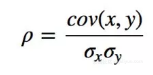Pearson相关系数的取值范围在-1到1之间，0代表无相关性，正数代表正相关，负数代表负相关，绝对值越大，相关性越高。

np.corrcoef(height, weight)[0,1] # 计算Pearson相关系数

0.47180417408477093

# 绘制身高、体重的散点图
plt.plot(height,weight, marker='.', linestyle='none', alpha = 0.05)
plt.xlabel('height (m)')
plt.ylabel('weight (kg)')
plt.title('correlation of weight and height')
plt.show()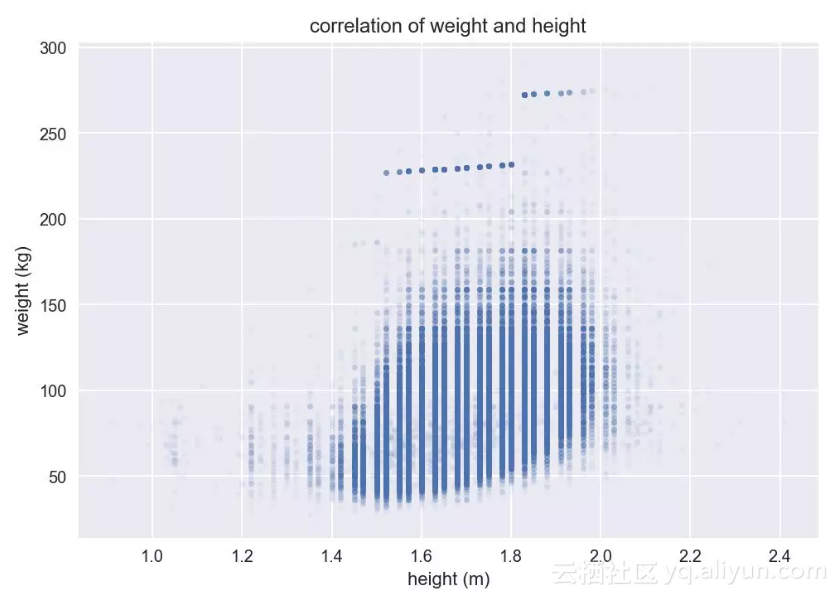# 计算BMI值和体重的相关系数
corr = np.corrcoef(weight, bmi)
print('Pearson correlation coefficient: %.2f' % corr[0,1] )

# 绘制BMI值和体重的散点图
plt.plot(weight,bmi, marker='.', linestyle='none', alpha = 0.05)
plt.xlabel('weight (kg)')
plt.ylabel('BMI')
plt.title('correlation of weight and BMI')
plt.show()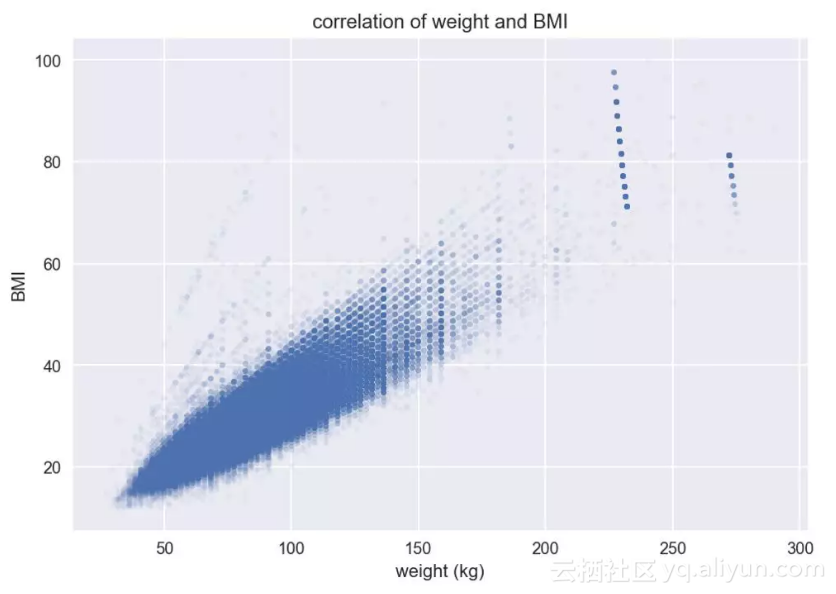BMI-体重散点图

# 计算BMI值和身高的相关系数
corr = np.corrcoef(height, bmi)
print('Pearson correlation coefficient: %.4f' % corr[0,1] )

# 绘制BMI值和身高的散点图
plt.plot(height,bmi, marker='.', linestyle='none', alpha = 0.05)
plt.xlabel('height (m)')
plt.ylabel('BMI')
plt.title('correlation of BMI and height')
plt.show(

Pearson correlation coefficient: -0.0060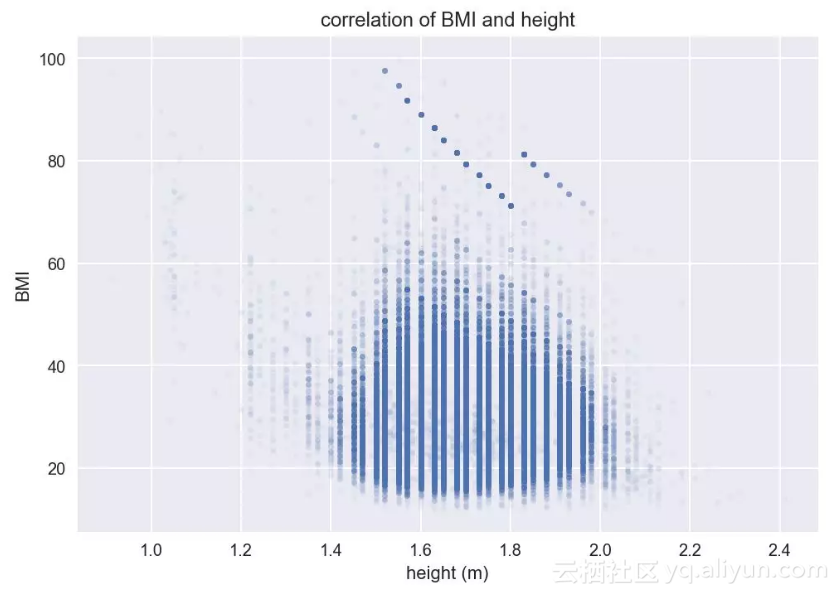BMI-身高散点图

●  开篇：数据分析流程
●  发掘数据中的信息 - 描述性统计分析（本文）
●  统计分布
●  参数估计

●  假设检验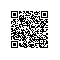Python爱好者使用钉钉扫一扫加入圈子
+ 订阅